Open in App
Not now

# Java 8 Stream Tutorial

• Difficulty Level : Easy
• Last Updated : 25 Jan, 2023

Introduced in Java 8, the Stream API is used to process collections of objects. A stream is a sequence of objects that supports various methods which can be pipelined to produce the desired result. Before proceeding further let us discuss out the difference between Collection and Streams in order to understand why this concept was introduced.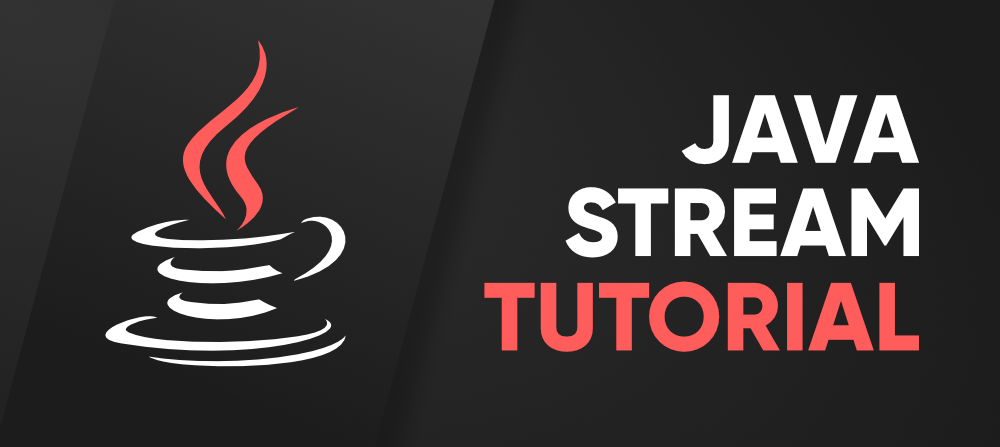Note:

• If we want to represent a group of objects as a single entity then we should go for collection.
• But if we want to process objects from the collection then we should go for streams.

If we want to use the concept of streams then stream() is the method to be used. Stream is available as an interface.

`Stream s = c.stream();`

In the above pre-tag, ‘c’ refers to the collection. So on the collection, we are calling the stream() method and at the same time, we are storing it as the Stream object. Henceforth, this way we are getting the Stream object.

Note: Streams are present in java’s utility package named java.util.stream

Let us now start with the basic components involved in streams. They as listed and as follows:

• Sequence of Elements
• Source
• Aggregate Operations
• Pipelining
• Internal iteration

Features of Java stream?

• A stream is not a data structure instead it takes input from the Collections, Arrays, or I/O channels.
• Streams don’t change the original data structure, they only provide the result as per the pipelined methods.
• Each intermediate operation is lazily executed and returns a stream as a result, hence various intermediate operations can be pipelined. Terminal operations mark the end of the stream and return the result.

Before moving ahead in the concept consider an example in which we are having ArrayList of integers, and we suppose we apply a filter to get only even numbers from the object inserted.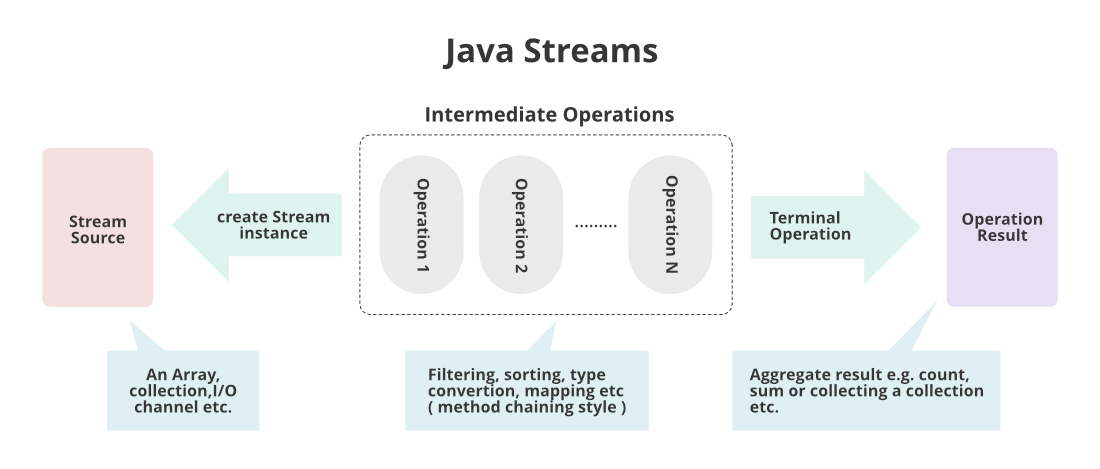How does Stream Work Internally?

In streams,

• To filter out from the objects we do have a function named filter()
• To impose a condition we do have a logic of predicate which is nothing but a functional interface. Here function interface can be replaced by a random expression. Hence, we can directly impose the condition check-in our predicate.
• To collect elements we will be using Collectors.toList() to collect all the required elements.
• Lastly, we will store these elements in a List and display the outputs on the console.

Example

## Java

 `// Java Program to illustrate FILTER & COLLECT Operations` `// Importing input output classes``import` `java.io.*;` `// Importing utility class for List and ArrayList classes``import` `java.util.*;` `// Importing stream classes``import` `java.util.stream.*;` `// Main class``public` `class` `GFG {` `    ``// Main driver method``    ``public` `static` `void` `main(String[] args)``    ``{` `        ``// Creating an ArrayList object of integer type``        ``ArrayList al = ``new` `ArrayList();` `        ``// Inserting elements to ArrayList class object``        ``// Custom input integer numbers``        ``al.add(``2``);``        ``al.add(``6``);``        ``al.add(``9``);``        ``al.add(``4``);``        ``al.add(``20``);` `        ``// First lets print the collection``        ``System.out.println(``"Printing the collection : "``                           ``+ al);` `        ``// Printing new line for better output readability``        ``System.out.println();` `        ``// Stream operations``        ``// 1. Getting the stream from this collection``        ``// 2. Filtering out only even elements``        ``// 3. Collecting the required elements to List``        ``List ls``            ``= al.stream()``                  ``.filter(i -> i % ``2` `== ``0``)``                  ``.collect(Collectors.toList());` `        ``// Print the collection after stream operation``        ``// as stored in List object``        ``System.out.println(``            ``"Printing the List after stream operation : "``            ``+ ls);``    ``}``}`

Output

```Printing the collection : [2, 6, 9, 4, 20]

Printing the List after stream operation : [2, 6, 4, 20]```

Output explanation: In our collection object, we were having elements entered using the add() operation. After processing the object in which they were stored through streams we impose a condition in the predicate of streams to get only even elements, we get elements in the object as per our requirement.  Hence, streams helped us this way in processing over-processed collection objects.

Various core operations over Streams?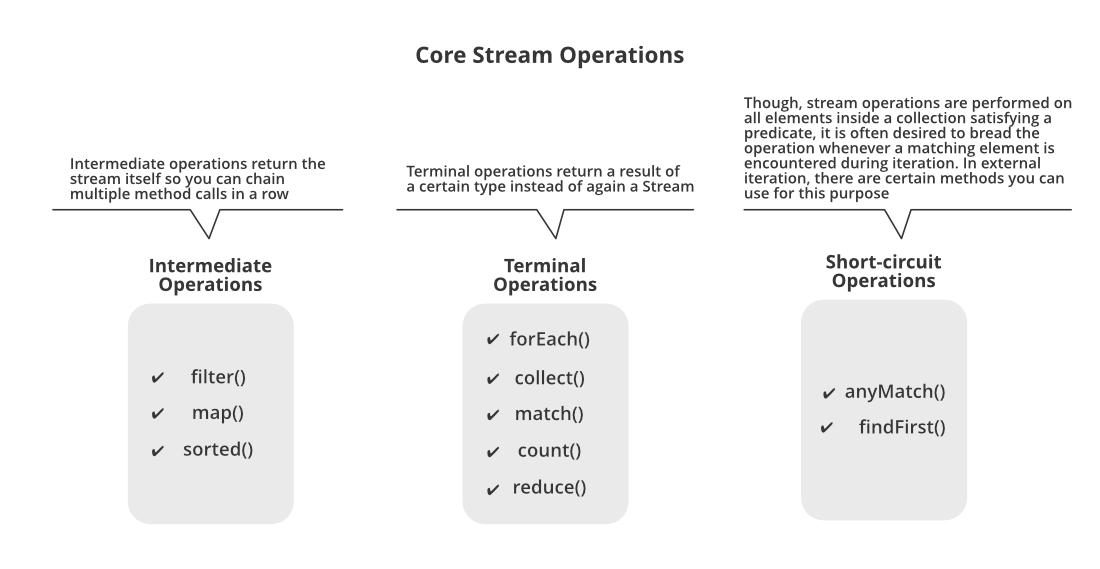There are broadly 3 types of operations that are carried over streams namely as follows as depicted from the image shown above:

1. Intermediate operations
2. Terminal operations
3. Short-circuit operations

Let us do discuss out intermediate operations here only in streams to a certain depth with the help of an example in order to figure out other operations via theoretical means. So, there are 3 types of Intermediate operations which are as follows:

• Operation 1: filter() method
• Operation 2: map() method
• Operation 3: sorted() method

All three of them are discussed below as they go hand in hand in nearly most of the scenarios and to provide better understanding by using them later by implementing in our clean java programs below. As we already have studied in the above example of which we are trying to filter processed objects can be interpreted as filter() operation operated over streams. Later on from that processed filtered elements of objects, we are collecting the elements back to List using Collectors for which we have imported a specific package named java.util.stream with the help of Collectors.toList() method. This is referred to as collect() operation in streams so here again we won’t be taking an example to discuss them out separately.

Example:

## Java

 `// Java program to illustrate Intermediate Operations``// in Streams` `// Importing required classes``import` `java.io.*;``import` `java.util.*;``import` `java.util.stream.*;` `// Main class``class` `Test {` `    ``// Main driver method``    ``public` `static` `void` `main(String[] args)``    ``{` `        ``// Creating an integer Arraylist to store marks``        ``ArrayList marks = ``new` `ArrayList();` `        ``// These are marks of the students``        ``// Considering 5 students so input entries``        ``marks.add(``30``);``        ``marks.add(``78``);``        ``marks.add(``26``);``        ``marks.add(``96``);``        ``marks.add(``79``);` `        ``// Printing the marks of the students before grace``        ``System.out.println(``            ``"Marks of students before grace : "` `+ marks);` `        ``// Now we want to grace marks by 6``        ``// using the streams to process over processing``        ``// collection` `        ``// Using stream, we map every object and later``        ``// collect to List``        ``// and store them``        ``List updatedMarks``            ``= marks.stream()``                  ``.map(i -> i + ``6``)``                  ``.collect(Collectors.toList());` `        ``// Printing the marks of the students after grace``        ``System.out.println(``            ``"Marks of students  after grace : "``            ``+ updatedMarks);``    ``}``}`

Output

```Marks of students before grace : [30, 78, 26, 96, 79]
Marks of students  after grace : [36, 84, 32, 102, 85]```

Note: For every object if there is urgency to do some operations be it square, double or any other than only we need to use map() function  operation else try to use filter() function operation.

Now geeks you are well aware of ‘why’ streams were introduced, but you should be wondering out ‘where’ to use it. The answer is very simple as we do use them too often in our day-to-day life. Hence, the geek in simpler words we say directly land p on wherever the concept of the collection is applicable, streams concept can be applied there.

### Real-life Example

Example 1: In general, daily world, whenever the data is fetching from the database, it is more likely we will be using collection so there itself streams concept is must apply to deal with processed data.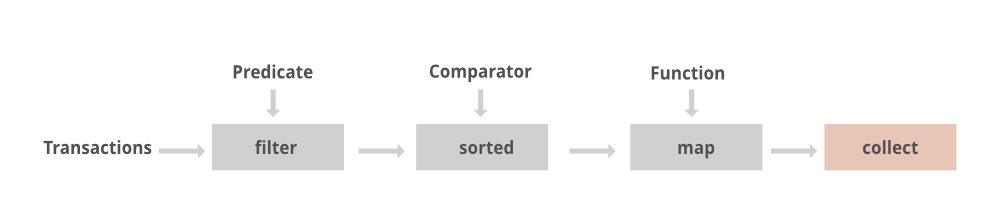Now we will be discussing out real-time examples to interrelate streams in our life. Here we will be taking the most widely used namely as follows:

1. Streams in a Grocery store
2. Streams in mobile networking

Example 2: Streams in a Grocery store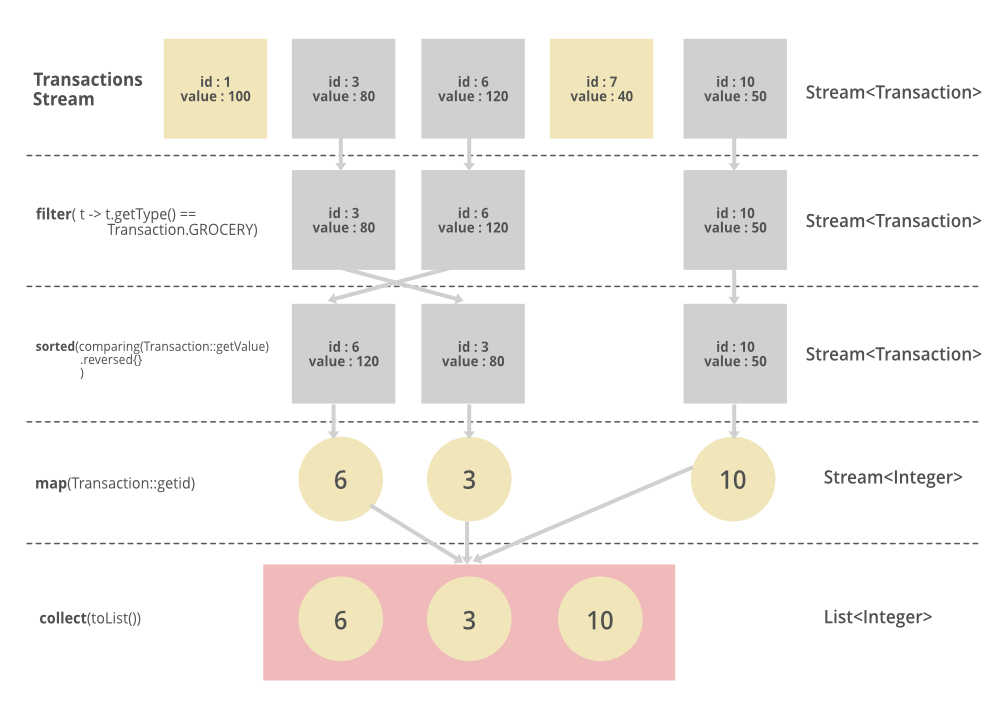The above pictorial image has been provided is implemented in streams which is as follows:

```List<Integer> transactionsIds =
transactions.stream()
.filter(t -> t.getType() == Transaction.GROCERY)
.sorted(comparing(Transaction::getValue).reversed())
.map(Transaction::getId)
.collect(toList());```

Example 3: Streams in mobile networking

Similarly, we can go for another widely used concept that is our dealing with our mobile numbers. Here we will not be proposing out listings, simply will be demonstrating how the stream concept is invoked in mobile networking by various service providers across the globe.

Collection can hold any number of object so let ‘mobileNumber’ be a collection and let it be holding various mobile numbers say it be holding 100+ numbers as objects. Suppose now the only carrier named ‘Airtel’ whom with which we are supposed to send a message if there is any migration between states in a country. So here streams concept is applied as if while dealing with all mobile numbers we will look out for this carrier using the filter() method operation of streams. In this way, we are able to deliver the messages without looking out for all mobile numbers and then delivering the message which senses impractical if done so as by now we are already too late to deliver. In this way these intermediate operations namely filter(), collect(), map() help out in the real world. Processing becomes super simpler which is the necessity of today’s digital world.

Hope by now you the users come to realize the power of streams in java as if we have to do the same task we do need to map corresponding to every object, increasing in code length, decreasing optimality of our code. With the usage of streams, we are able to in a single line irrespective of elements contained in the object as with the concept of streams we are dealing with the object itself.

Note: filter, sorted, and map, which can be connected together to form a pipeline.

My Personal Notes arrow_drop_up